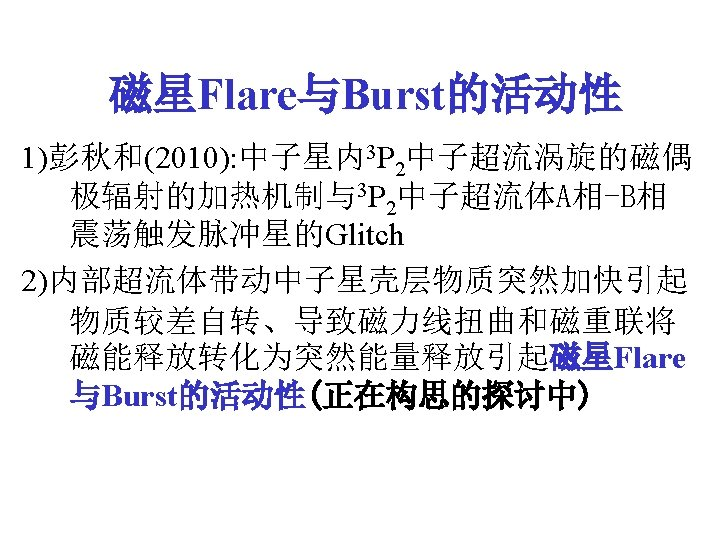# Relation of Electron Fermi Energy With Magnetic Field

• Slides: 44Relation of Electron Fermi Energy With Magnetic Field in Magnetars and their x-ray Luminosity Qiu-he Peng (Nanjing University )Some published relative works 1) Qiu-he Peng and Hao Tong, 2007, “The Physics of Strong magnetic fields in neutron stars”, Mon. Not. R. Astron. Soc. 378, 159 -162(2007) Observed strong magnetic fields (1011 -1013 gauss) is actually come from one induced by Pauli paramagnetic moment for relativistic degenerate electron gas 2) Qiu-he Peng & Hao Tong, 2009, “The Physics of Strong Magnetic Fields and Activity of Magnetars” Procedings of Science (Nuclei in the Cosmos X) 189, 10 th Symposium On Nuclei in the Cosmos, 27 July – 1 August 2008, Mackinac Island, Michigan, USA It is found that super strong magnetic fields of magnetars is come from one induced by paramagnetic moment for anisotropic 3 P neutron Cooper pairs 2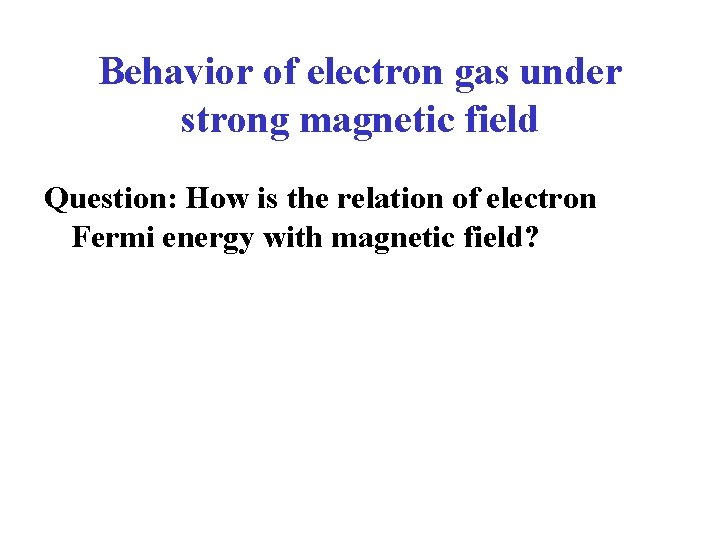Behavior of electron gas under strong magnetic field Question: How is the relation of electron Fermi energy with magnetic field?Landau Column under strong magnetic field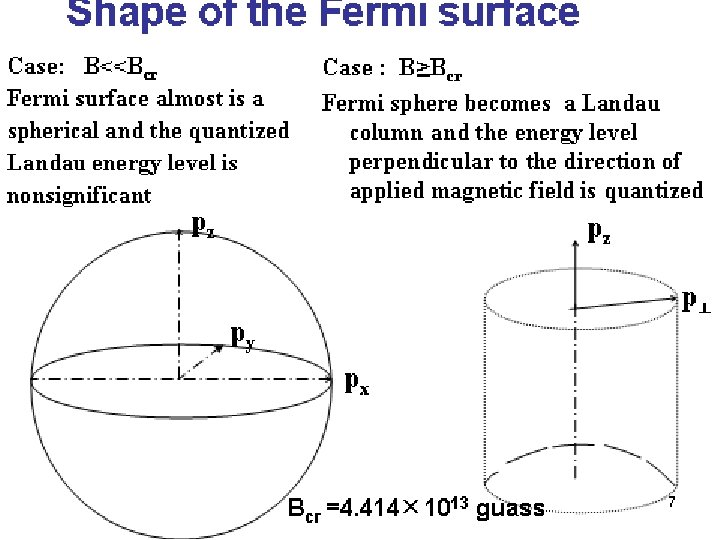pz n=6 n=5 n=4 n=3 n=2 n=1 n=0 Landau quantization p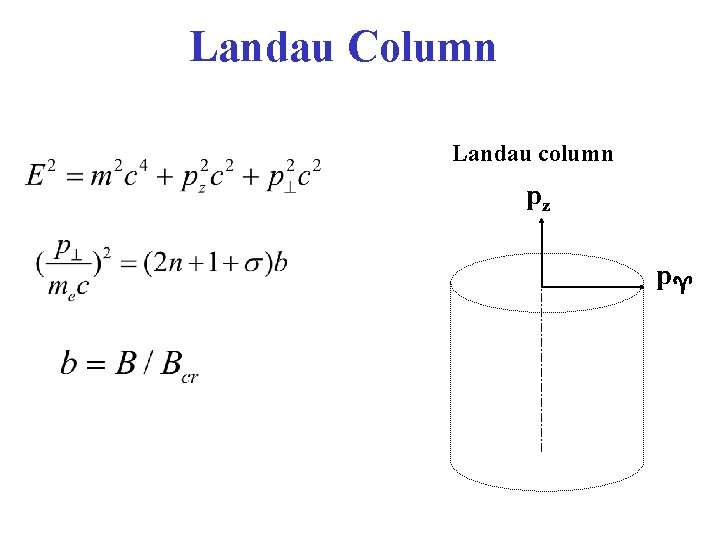Landau Column Landau column pz pIn the case B > Bcr (Landau coulumn) The overwhelming majority of neutrons congregates in the lowest levels n=0 or n=1, When The Landau column is a very long cylinder along the magnetic filed, but it is very narrow. The radius of its cross section is p . pz pFermi sphere in strong magnetic field: Fermi sphere without magnetic field : Both dpz and dp chang continuously. the microscopic state number in a volume element of phase space d 3 x d 3 p is d 3 x d 3 p /h 3. Fermi sphere in strong magnetic field: along the z-direction dpz changes continuously. In the x-y plane, electrons are populated on discrete Landau levels with n=0, 1, 2, 3… For a given pz (pz is still continuous)，there is a maximum orbital quantum number nmax(pz, b, σ)≈nmax(pz, b). In strong magnetic fields, an envelope of these Landau cycles with maximum orbital quantum number nmax(pz, b, σ) (0 pz p. F ) will approximately form a spherical sphere, i. e. Fermi sphere.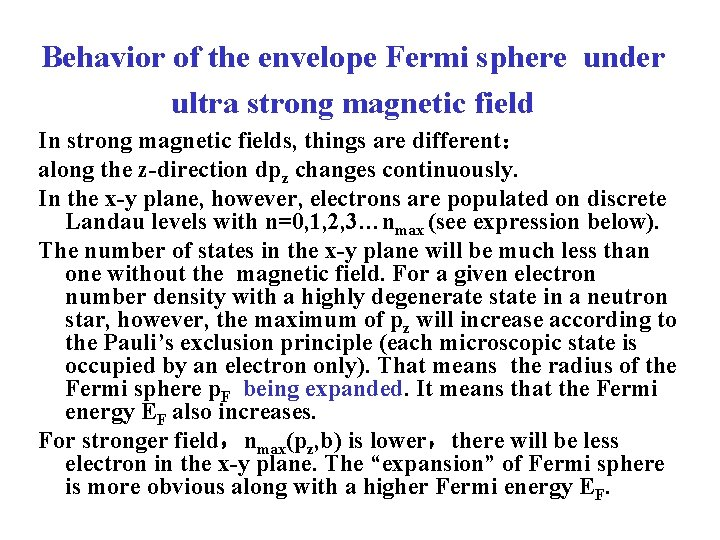Behavior of the envelope Fermi sphere under ultra strong magnetic field In strong magnetic fields, things are different： along the z-direction dpz changes continuously. In the x-y plane, however, electrons are populated on discrete Landau levels with n=0, 1, 2, 3…nmax (see expression below). The number of states in the x-y plane will be much less than one without the magnetic field. For a given electron number density with a highly degenerate state in a neutron star, however, the maximum of pz will increase according to the Pauli’s exclusion principle (each microscopic state is occupied by an electron only). That means the radius of the Fermi sphere p. F being expanded. It means that the Fermi energy EF also increases. For stronger field，nmax(pz, b) is lower，there will be less electron in the x-y plane. The “expansion” of Fermi sphere is more obvious along with a higher Fermi energy EF.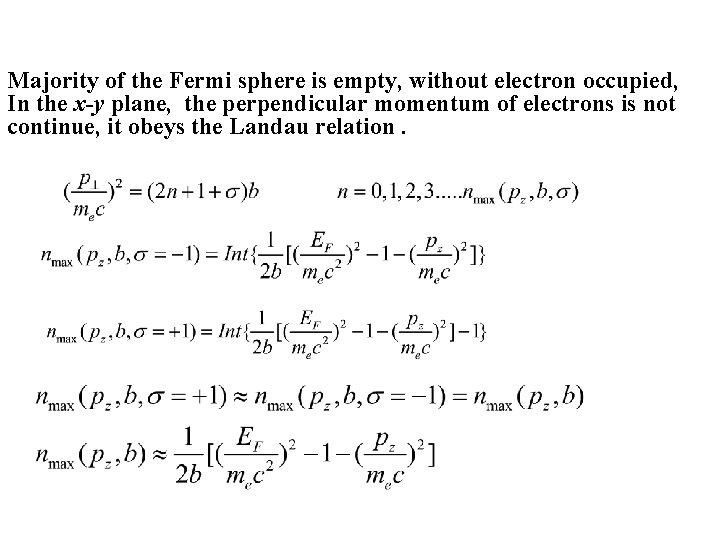Majority of the Fermi sphere is empty, without electron occupied, In the x-y plane, the perpendicular momentum of electrons is not continue, it obeys the Landau relation.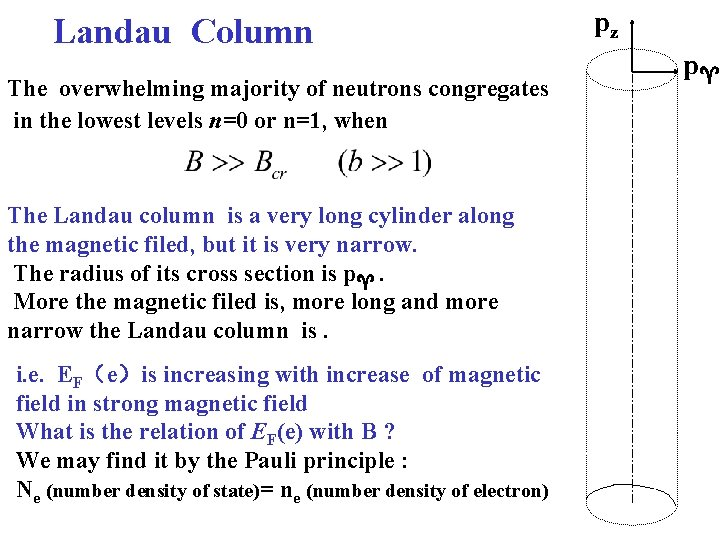Landau Column The overwhelming majority of neutrons congregates in the lowest levels n=0 or n=1, when The Landau column is a very long cylinder along the magnetic filed, but it is very narrow. The radius of its cross section is p . More the magnetic filed is, more long and more narrow the Landau column is. i. e. EF（e）is increasing with increase of magnetic field in strong magnetic field What is the relation of EF(e) with B ? We may find it by the Pauli principle : Ne (number density of state)= ne (number density of electron) pz pAn another popular theory Main idea: electron Fermi Energy decreases with increasing magnetic field Typical papers referenced by many papers in common a) Dong Lai, S. L. Shapiro, Ap. J. , 383(1991) 745 -761 b): Dong Lai, Matter in Strong Magnetic Fields (Reviews of Modern Physics>, 2001, 73: 629 -661) c) Harding & Lai , Physics of Strongly Magnetized Neutron Stars. (Rep. Prog. Phys. 69 (2006): 2631 -2708)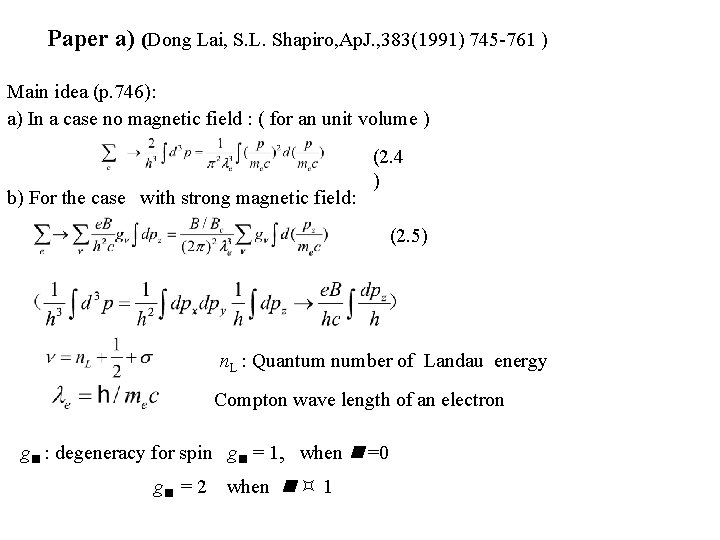Paper a) (Dong Lai, S. L. Shapiro, Ap. J. , 383(1991) 745 -761 ) Main idea (p. 746): a) In a case no magnetic field : ( for an unit volume ) b) For the case with strong magnetic field: (2. 4 ) (2. 5) n. L : Quantum number of Landau energy Compton wave length of an electron g : degeneracy for spin g = 1, when =0 g = 2 when 1Paper a): continue Number density of electrons in the case T → 0 (2. 6) It is the maximum of momentum of the electron along z-direction With a given quantum number (2. 7) e : chemical potential of electrons ( i. e. Fermi energy). The up limit, m , of the sum is given by the condition following (2. 8)Paper b): Matter in Strong Magnetic Fields (Reviews of Modern Physics>, 2001, 73: 629 -661) §VI. Free-Electron Gas in Strong Magnetic Fields (p. 647)中: The pressure of free electrons is isotropic. ρ0 is the radius of gyration of the electron in magnetic field Note 1: n. L in paper b) is in paper a) really Note 2: in the paper c) (Harding @Lai , 2006 Rrp. Prog. Phys. 69: 2631 -2708), (108)-(110) in § 6. 2 (p. 2669) are the same as (6. 1)-(6. 3) above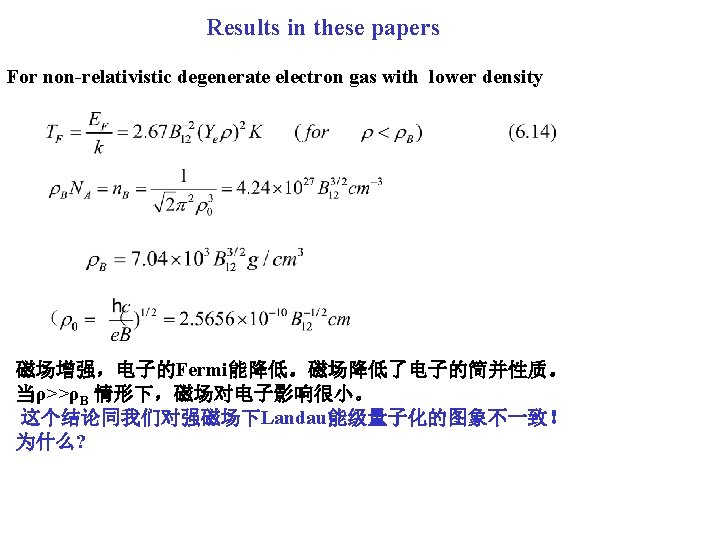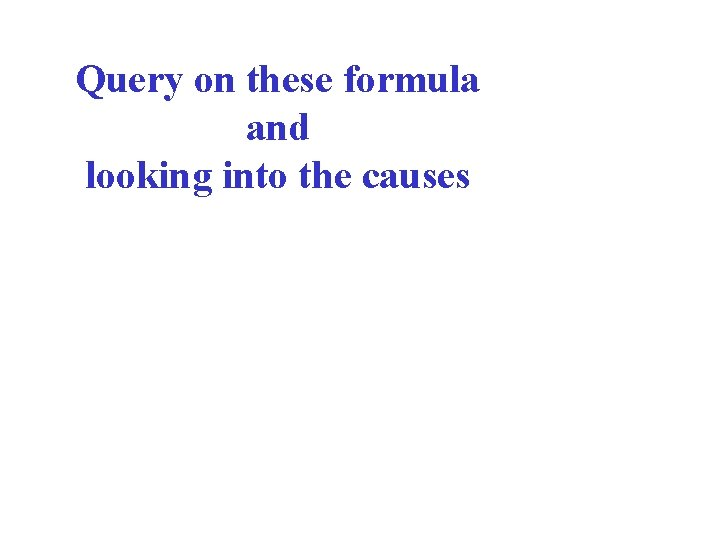Query on these formula and looking into the causes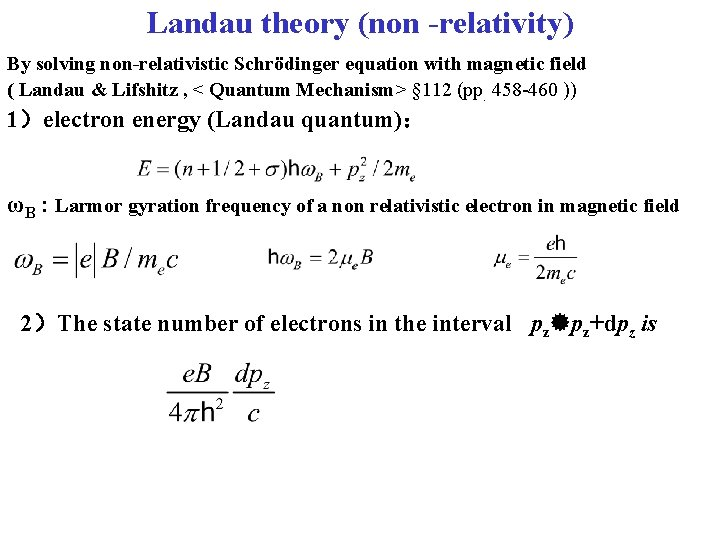Landau theory (non -relativity) By solving non-relativistic Schrödinger equation with magnetic field ( Landau & Lifshitz , < Quantum Mechanism> § 112 (pp. 458 -460 )) 1）electron energy (Landau quantum)： ωB : Larmor gyration frequency of a non relativistic electron in magnetic field 2）The state number of electrons in the interval pz pz+dpz isRelativistic Landau Energy in strong magnetic field In the case EF >>mec 2 , Landau energy is by solving the relativistic Dirac equation in magnetic field (Bohr magnetic moment of the electron) Landau quantum in strong magnetic field n: quantum number of the Landau energy level n=0, 1, 2, 3……(当n = 0 时, 只有σ= -1)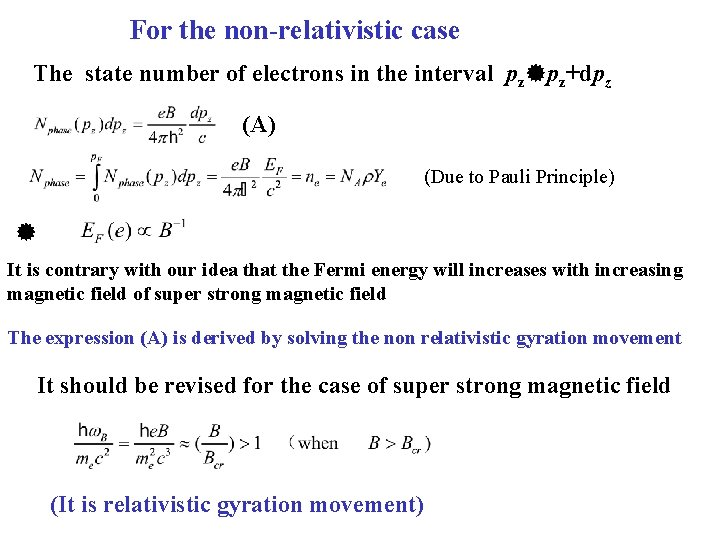For the non-relativistic case The state number of electrons in the interval pz pz+dpz (A) (Due to Pauli Principle) It is contrary with our idea that the Fermi energy will increases with increasing magnetic field of super strong magnetic field The expression (A) is derived by solving the non relativistic gyration movement It should be revised for the case of super strong magnetic field (It is relativistic gyration movement)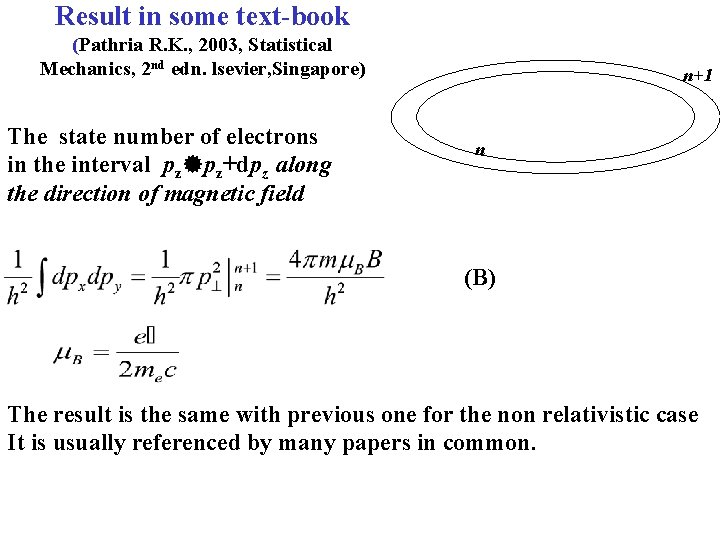Result in some text-book (Pathria R. K. , 2003, Statistical Mechanics, 2 nd edn. lsevier, Singapore) The state number of electrons in the interval pz pz+dpz along the direction of magnetic field n+1 n (B) The result is the same with previous one for the non relativistic case It is usually referenced by many papers in common.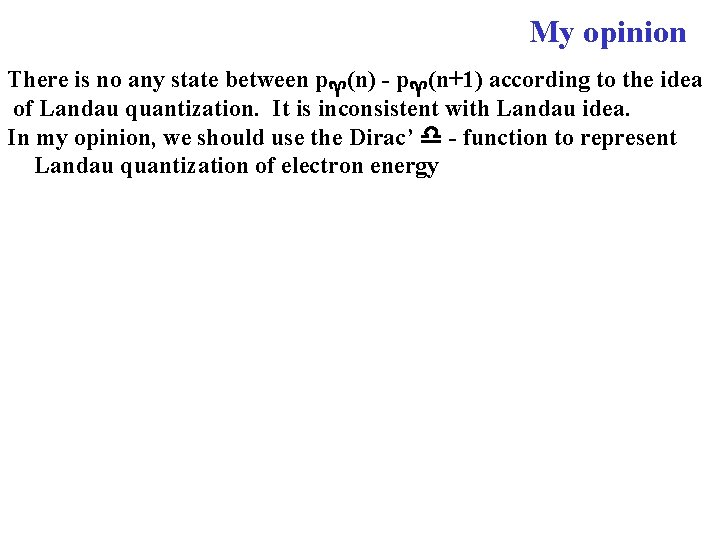My opinion There is no any state between p (n) - p (n+1) according to the idea of Landau quantization. It is inconsistent with Landau idea. In my opinion, we should use the Dirac’ - function to represent Landau quantization of electron energy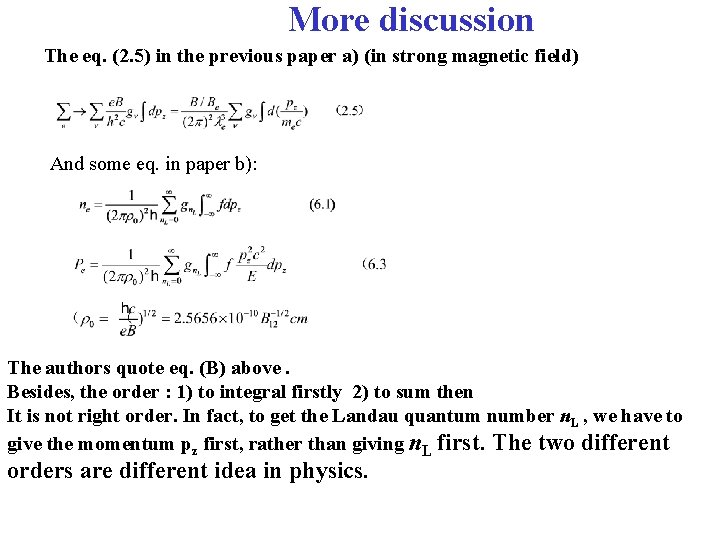More discussion The eq. (2. 5) in the previous paper a) (in strong magnetic field) And some eq. in paper b): The authors quote eq. (B) above. Besides, the order : 1) to integral firstly 2) to sum then It is not right order. In fact, to get the Landau quantum number n. L , we have to give the momentum pz first, rather than giving n. L first. The two different orders are different idea in physics.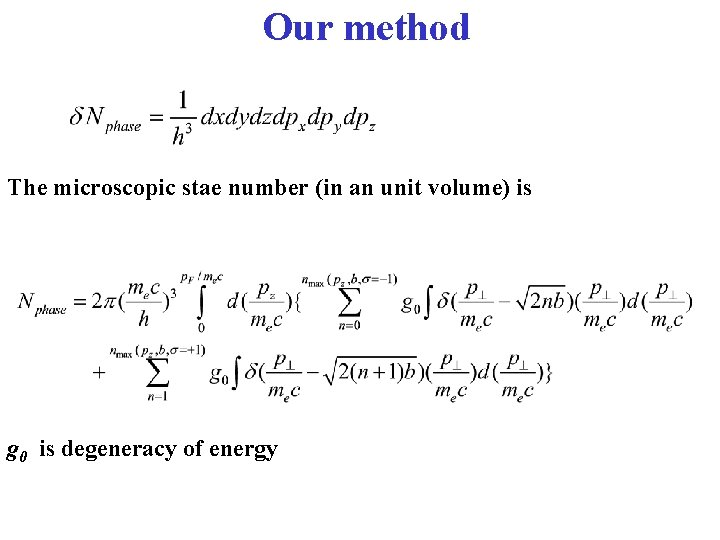Our method The microscopic stae number (in an unit volume) is g 0 is degeneracy of energy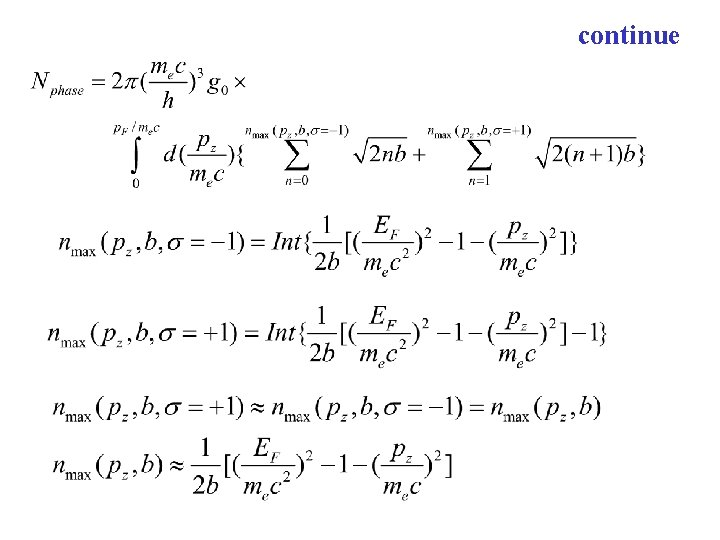continue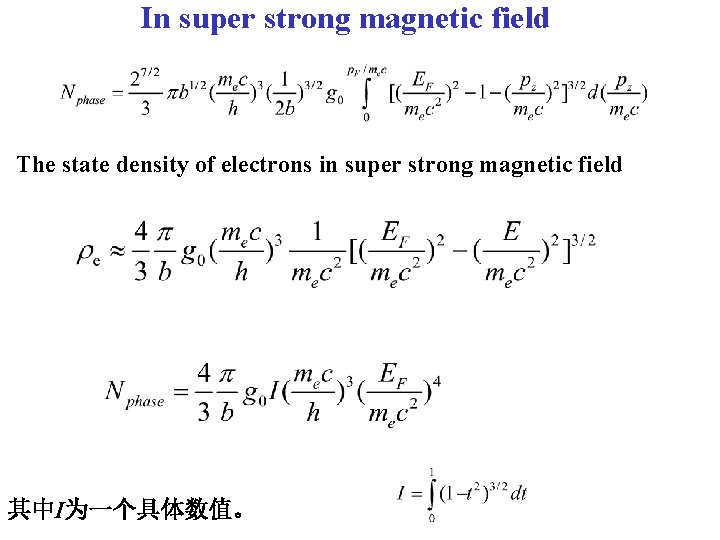In super strong magnetic field The state density of electrons in super strong magnetic field 其中I为一个具体数值。Principle of Pauli’s incompatibility Pauli principle: The total number states ( per unite volume) occupied by the electrons in the complete degenerate electron gas should be equal to the number density of the electrons.Relation between Fermi energy of electrons and magnetic field For the case with lower magnetic field in NS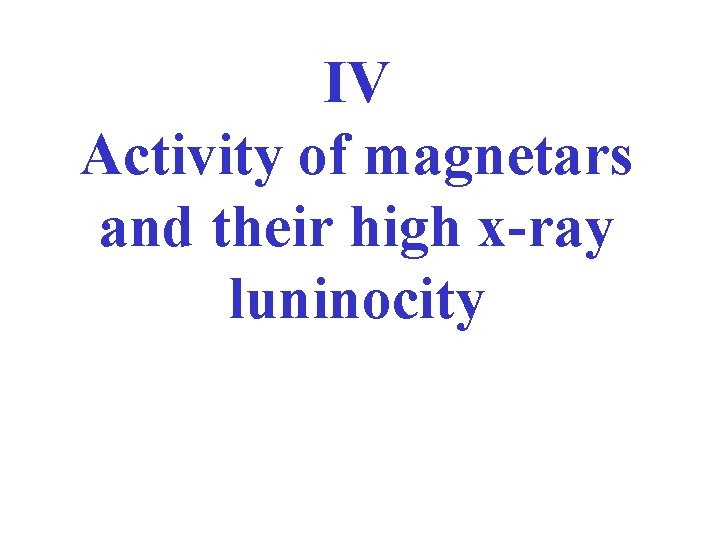IV Activity of magnetars and their high x-ray luninocity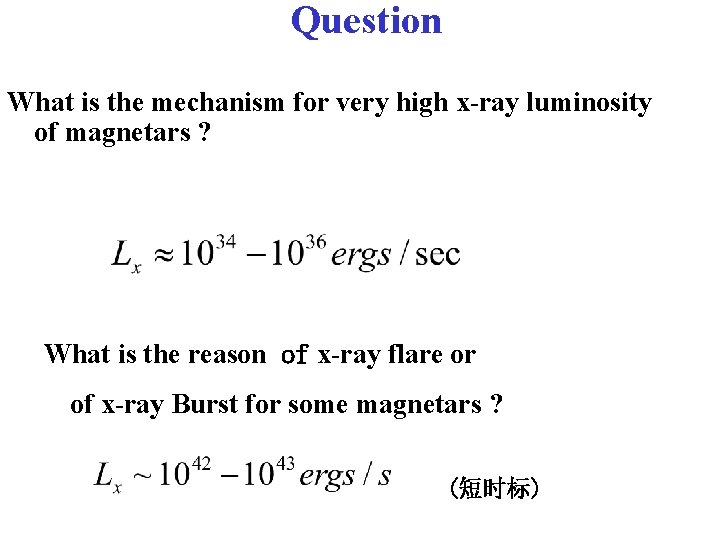Question What is the mechanism for very high x-ray luminosity of magnetars ? What is the reason of x-ray flare or of x-ray Burst for some magnetars ? (短时标)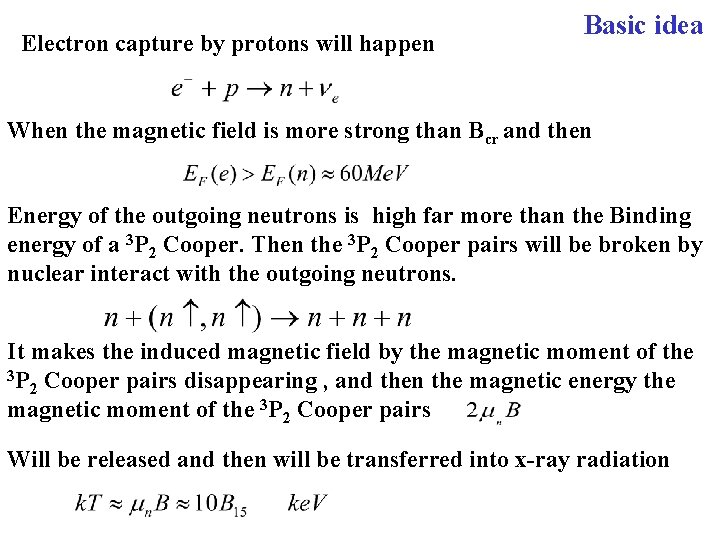Electron capture by protons will happen Basic idea When the magnetic field is more strong than Bcr and then Energy of the outgoing neutrons is high far more than the Binding energy of a 3 P 2 Cooper. Then the 3 P 2 Cooper pairs will be broken by nuclear interact with the outgoing neutrons. It makes the induced magnetic field by the magnetic moment of the 3 P Cooper pairs disappearing , and then the magnetic energy the 2 magnetic moment of the 3 P 2 Cooper pairs Will be released and then will be transferred into x-ray radiationTotal Energy may be released It may take ~ 104 -106 yr for x-ray luminocity of AXPs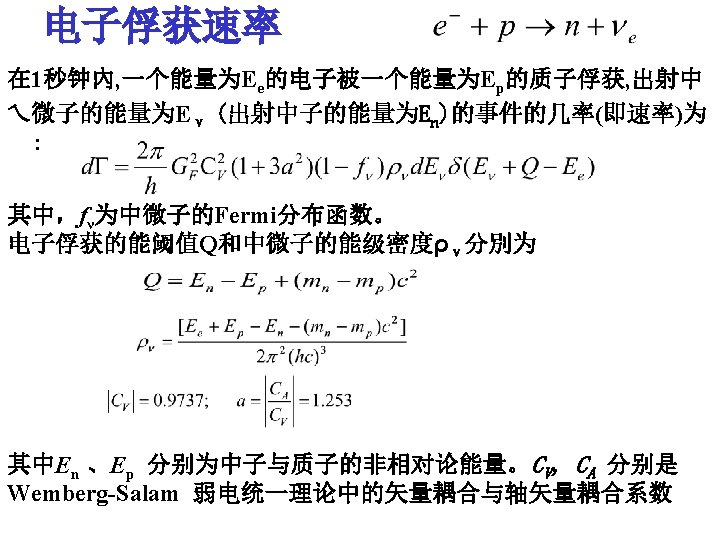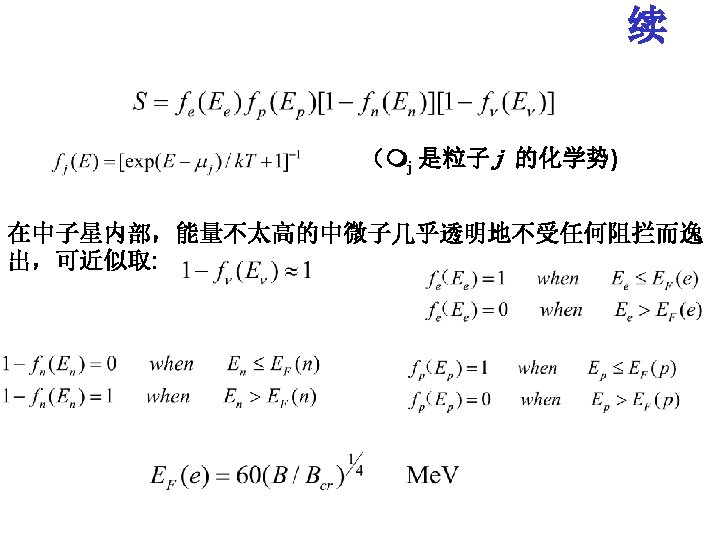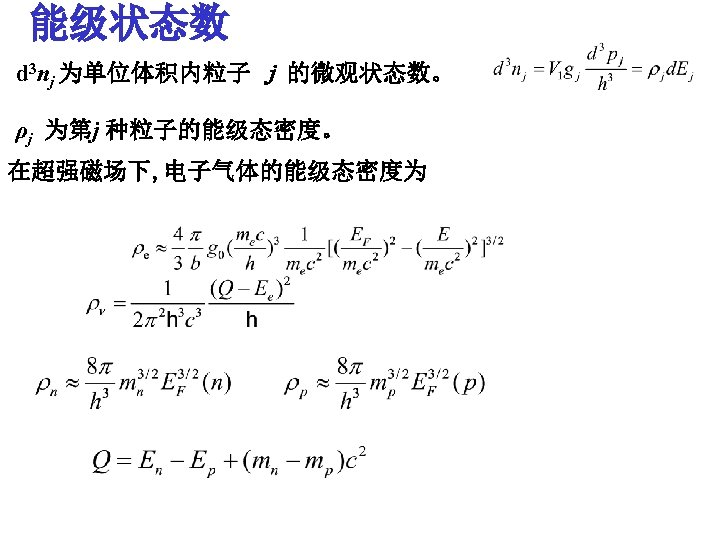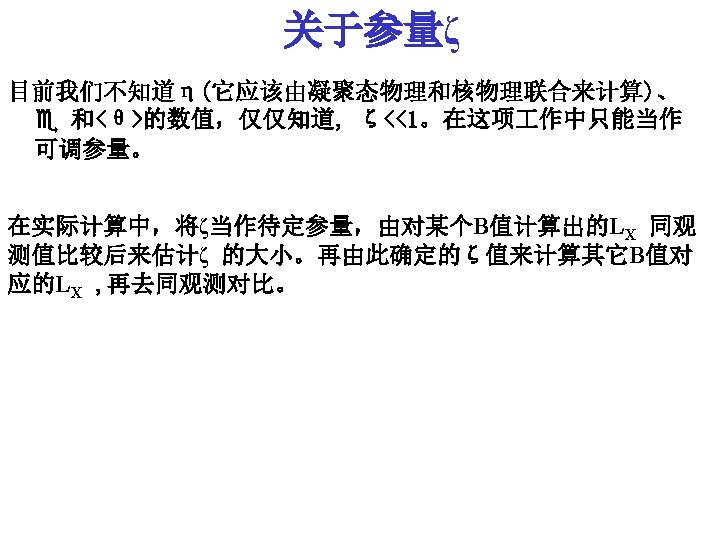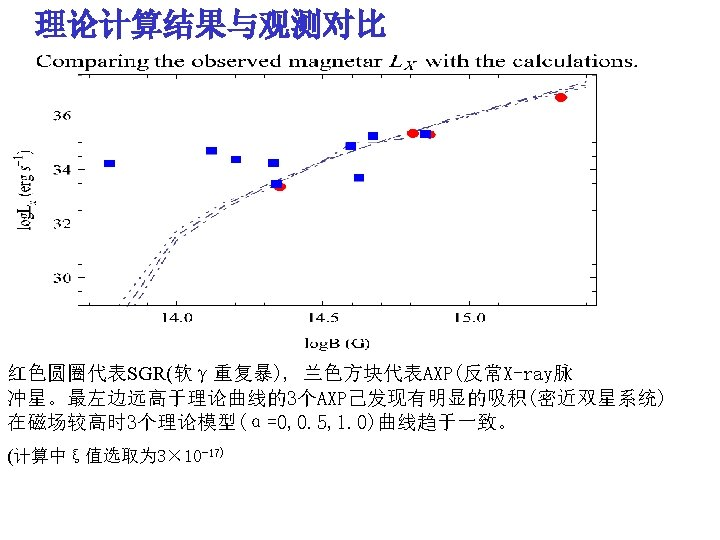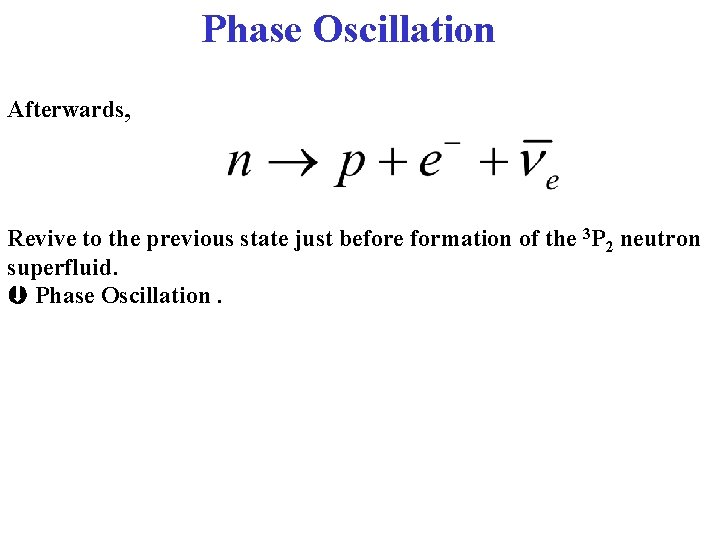Phase Oscillation Afterwards, Revive to the previous state just before formation of the 3 P 2 neutron superfluid. Phase Oscillation.Questions? 1. Detail process: The rate of the process ÞTime scale ? ? 2. What is the real maximum magnetic field of the magnetars? 3. How long is the period of oscillation above? 4. How to compare with observational data 5. Estimating the appearance frequency of AXP and SGR ?